Students can download Maths Chapter 3 Algebra Ex 3.16 Questions and Answers, Notes, Samacheer Kalvi 10th Maths Guide Pdf helps you to revise the complete Tamilnadu State Board New Syllabus, helps students complete homework assignments and to score high marks in board exams.

## Tamilnadu Samacheer Kalvi 10th Maths Solutions Chapter 3 Algebra Ex 3.16

1. In the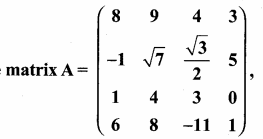write (i) The number of elements
(ii) The order of the matrix
(iii) Write the elements a22, a23, a24, a34, a43, a44.
(i) The number of elements is 16
(ii) The order of the matrix is 4 × 4
(iii) Elements corresponds to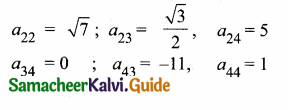Question 2.
If a matrix has 18 elements, what are the possible orders it can have? What if it has 6 elements?
The possible orders of the matrix having 18 elements are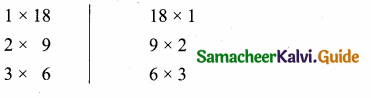The possible orders of the matrix having 6 elements are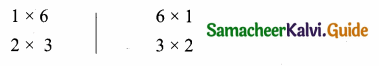Question 3.
Construct a 3 × 3 matrix whose elements are given by
(i) aij = |i – 2j|
aij = |i – 2j|
The general 3 × 3 matrices is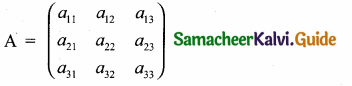a11 = |1 – 2(1)| = |1 – 2| = | – 1| = 1
a12 = |1 – 2(2)| = |1 – 4| = | – 3| = 3
a13 = |1 – 2(3)| = |1 – 6| = | – 5| = 5
a21 = |2 – 2(1)| = |2 – 2| = 0 = 0
a22 = |2 – 2(2)| = |2 – 4| = | – 2| = 2
a23 = |2 – 2(3)| = |2 – 6| = | – 4| = 4
a31 = |3 – 2(1)| = |3 – 2| = | 1 | = 1
a32 = |3 – 2(2)| = |3 – 4| = | – 1 | = 1
a33 = |3 – 2(3)| = |3 – 6| = | – 3 | = 3
The required matrix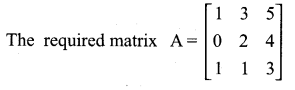(ii) aij = $$\frac{(i+j)^{3}}{3}$$
a11 = $$\frac{(1+1)^{3}}{3}$$ = $$\frac{2^{3}}{3}$$ = $$\frac { 8 }{ 3 }$$
a12 = $$\frac{(1+2)^{3}}{3}$$ = $$\frac { 27 }{ 3 }$$ = 9
a13 = $$\frac{(1+3)^{3}}{3}$$ = $$\frac { 64 }{ 3 }$$ = $$\frac { 64 }{ 3 }$$
a21 = $$\frac{(2+1)^{3}}{3}$$ = $$\frac { 27 }{ 3 }$$ = 9
a22 = $$\frac{(2+2)^{3}}{3}$$ = $$\frac { 64 }{ 3 }$$ = $$\frac { 64 }{ 3 }$$
a23 = $$\frac{(2+3)^{3}}{3}$$ = $$\frac { 125 }{ 3 }$$ = $$\frac { 125 }{ 3 }$$
a31 = $$\frac{(3+1)^{3}}{3}$$ = $$\frac { 64 }{ 3 }$$ = $$\frac { 64 }{ 3 }$$
a32 = $$\frac{(3+2)^{3}}{3}$$ = $$\frac { 125 }{ 3 }$$ = $$\frac { 125 }{ 3 }$$
a33 = $$\frac{(3+3)^{3}}{3}$$ = $$\frac { 216 }{ 3 }$$ = 72
The required matrixQuestion 4.
Ifthen find the tranpose of A.transpose of A = (AT)Question 5.
Ifthen find the tranpose of – A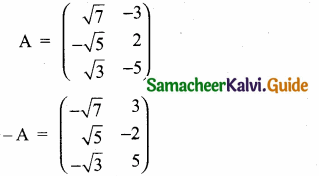Transpose of – A = (-AT) =Question 6.
If A =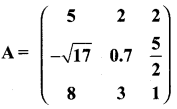then verify (AT)T = AHence it is verified

Question 7.
Find the values of x, y and z from the following equations
(i)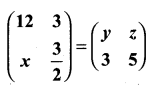Since the given matrices are equal then all the corresponding elements are equal.
y = 12, z = 3, x = 3
The value of x = 3, y = 12 and z = 3(ii)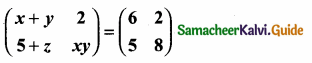x + y = 6 ……(1)
5 + z = 5
z = 5 – 5 = 0
xy = 8
y = $$\frac { 8 }{ x }$$
Substitute the value of y = $$\frac { 8 }{ x }$$ in (1)
x + $$\frac { 8 }{ x }$$ = 6
x2 + 8 = 6x
x2 – 6x + 8 = 0
(x – 4) (x – 2) = 0
∴ x – 4 = 0 or x – 2 = 0
x = 4 or x = 2
y = $$\frac { 8 }{ 4 }$$ = 2 or y = $$\frac { 8 }{ 2 }$$ = 4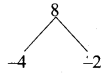∴ The value of x, y and z are 4, 2, 0 (or) 2, 4, 0(iii)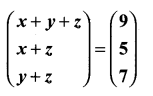Solution:
x + y + z = 9 ……….(1)
x + z = 5 ……….(2)
y + z = 7 ……….(3)Substitute the value of y = 4 in (3)
y + z = 7
4 + z = 7
z = 7 – 4
= 3
Substitute the value of z = 3 in (2)
x + 3 = 5
x = 5 – 3
= 2
∴ The value of x = 2 , y = 4 and z = 3